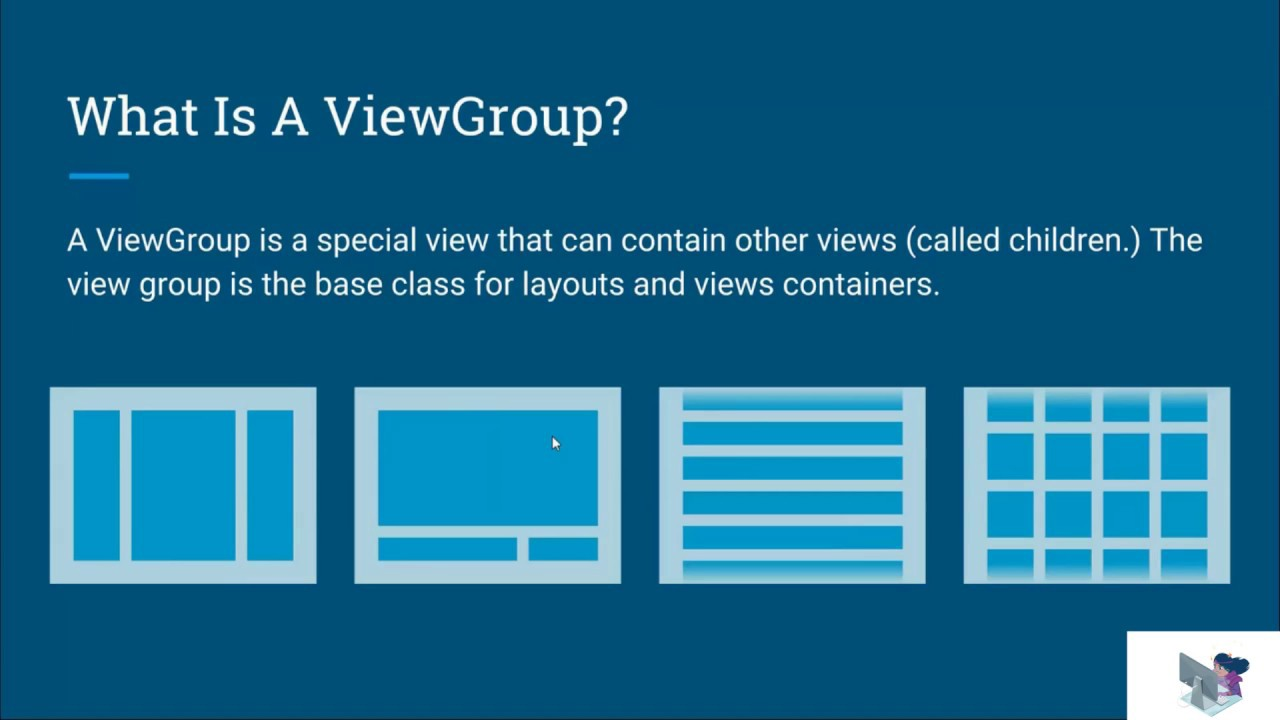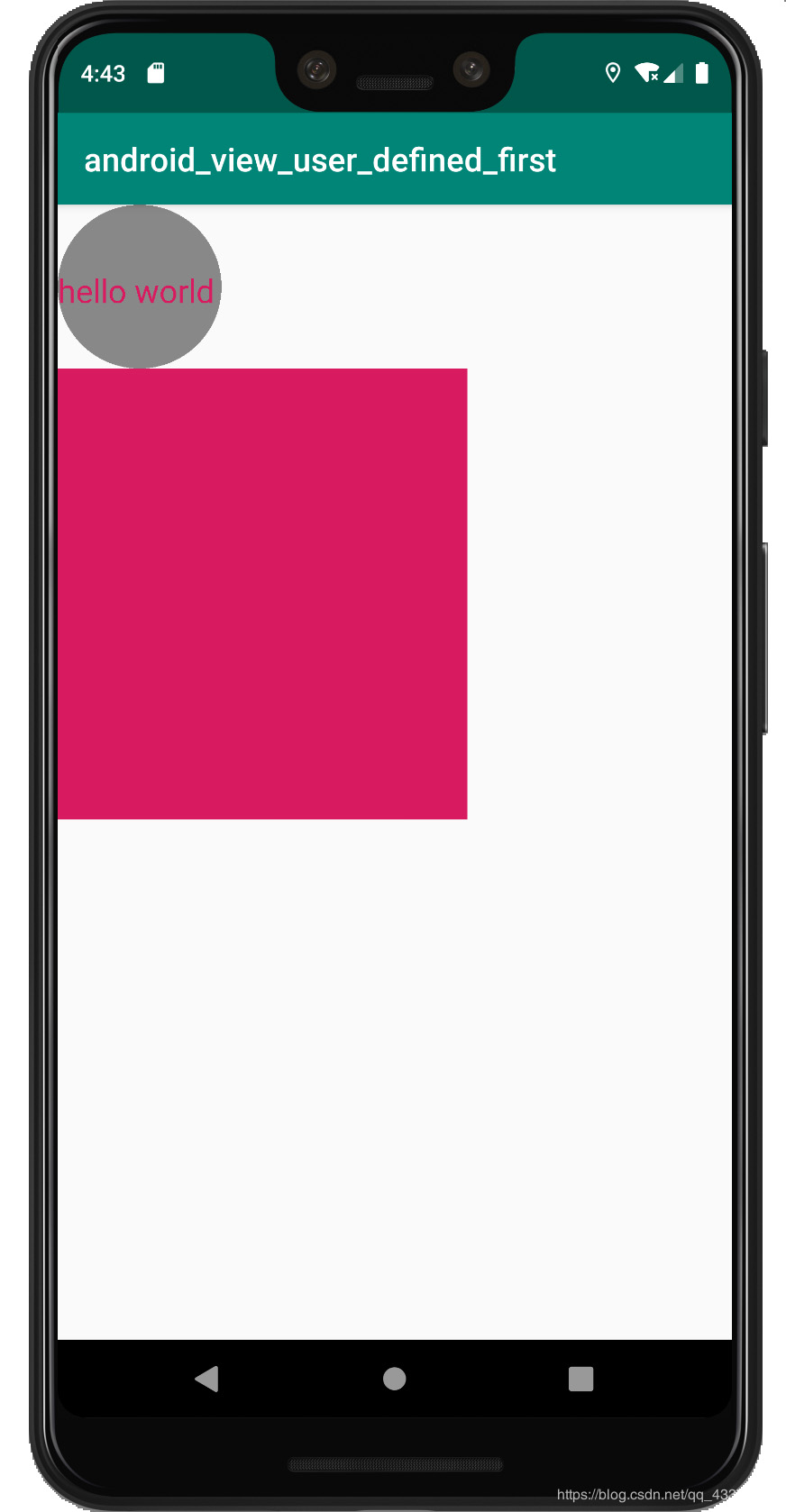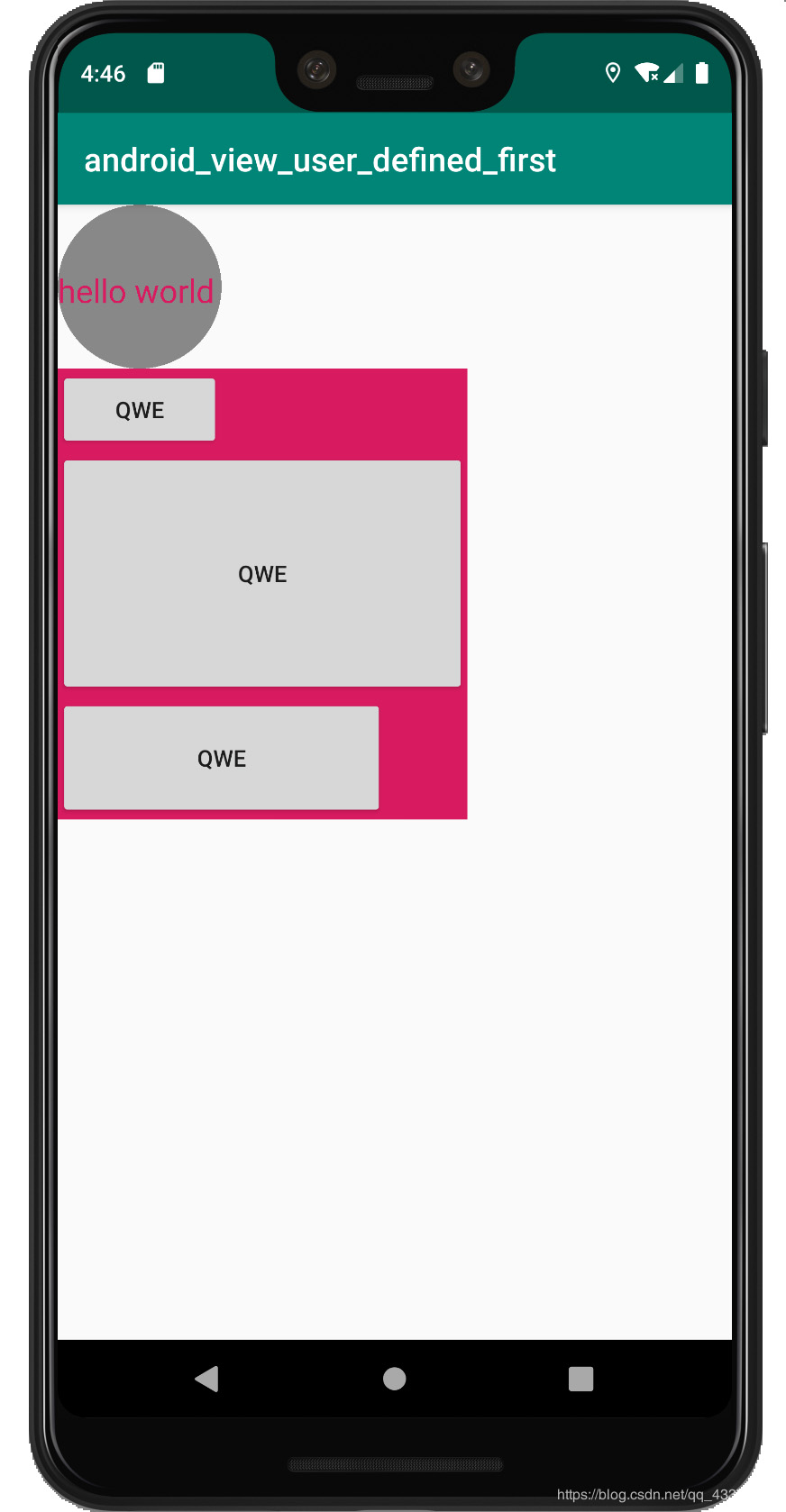# Android進階教程之ViewGroup自定義布局

更新時間：2019年06月23日 09:15:02   作者：水中魚之1999我要評論``` private int getMaxWidth(){
int count = getChildCount();
int maxWidth = 0;
for (int i = 0 ; i < count ; i ++){
int currentWidth = getChildAt(i).getMeasuredWidth();
if (maxWidth < currentWidth){
maxWidth = currentWidth;
}
}
return maxWidth;
}

private int getTotalHeight(){
int count = getChildCount();
int totalHeight = 0;
for (int i = 0 ; i < count ; i++){
totalHeight += getChildAt(i).getMeasuredHeight();
}
}```

``` @Override
protected void onMeasure(int widthMeasureSpec, int heightMeasureSpec) {
super.onMeasure(widthMeasureSpec, heightMeasureSpec);

measureChildren(widthMeasureSpec, heightMeasureSpec);

int widthMode = MeasureSpec.getMode(widthMeasureSpec);
int width  = MeasureSpec.getSize(widthMeasureSpec);
int heightMode= MeasureSpec.getMode(heightMeasureSpec);
int height = MeasureSpec.getSize(heightMeasureSpec);

if (widthMode == MeasureSpec.AT_MOST && heightMode == MeasureSpec.AT_MOST){
int groupWidth = getMaxWidth();
int groupHeight= getTotalHeight();

setMeasuredDimension(groupWidth, groupHeight);
}else if (widthMode == MeasureSpec.AT_MOST){
setMeasuredDimension(getMaxWidth(), height);
}else if (heightMode == MeasureSpec.AT_MOST){
setMeasuredDimension(width, getTotalHeight());
}
}```

``` <com.entry.android_view_user_defined_first.views.MyLinearLayout
android:layout_width="wrap_content"
android:layout_height="wrap_content"
android:background="@color/colorAccent">

<Button
android:layout_width="100dp"
android:layout_height="50dp"
android:text="qwe"/>

<Button
android:layout_width="250dp"
android:layout_height="150dp"
android:text="qwe"/>

<Button
android:layout_width="200dp"
android:layout_height="75dp"
android:text="qwe"/>

</com.entry.android_view_user_defined_first.views.MyLinearLayout>`````` @Override
protected void onLayout(boolean changed, int l, int t, int r, int b) {
int count = getChildCount();
int currentHeight = 0;
for (int i = 0 ; i < count ; i++){
View view = getChildAt(i);
int height = view.getMeasuredHeight();
int width = view.getMeasuredWidth();
view.layout(l, currentHeight, l + width, currentHeight + height);
currentHeight += height;
}
}```